# Algebraic systems, quasi-variety of

(diff) ← Older revision | Latest revision (diff) | Newer revision → (diff)

A class of algebraic systems (-systems) axiomatized by special formulas of a first-order logical language, called quasi-identities or conditional identities, of the form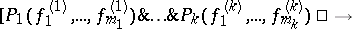where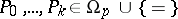, and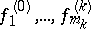are terms of the signaturein the object variables. By virtue of Mal'tsev's theorem  a quasi-varietyof algebraic systems of signaturecan also be defined as an abstract class of-systems containing the unit-system, and which is closed with respect to subsystems and filtered products , . An axiomatizable class of-systems is a quasi-variety if and only if it contains the unit-system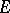and is closed with respect to subsystems and Cartesian products. If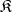is a quasi-variety of signature, the subclass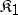of systems ofthat are isomorphically imbeddable into suitable systems of some quasi-variety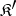with signature, is itself a quasi-variety. Thus, the class of semi-groups imbeddable into groups is a quasi-variety; the class of associative rings without zero divisors imbeddable into associative skew-fields is also a quasi-variety.

A quasi-varietyof signatureis called finitely definable (or, is said to have a finite basis of quasi-identities) if there exists a finite setof quasi-identities ofsuch thatconsists of only those-systems in which all the formulas from the setare true. For instance, the quasi-variety of all semi-groups with cancellation is defined by the two quasi-identities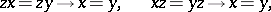and is therefore finitely definable. On the other hand, the quasi-variety of semi-groups imbeddable into groups has no finite basis of quasi-identities , .

Letbe an arbitrary (not necessarily abstract) class of-systems; the smallest quasi-variety containingis said to be the implicative closure of the class. It consists of subsystems of isomorphic copies of filtered products of-systems of the class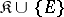, whereis the unit-system. Ifis the implicative closure of a classof-systems,is called a generating class of the quasi-variety. A quasi-varietyis generated by one system if and only if for any two systems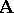,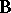ofthere exists in the classa system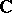containing subsystems isomorphic toand. Any quasi-varietycontaining systems other than one-element systems has free systems of any rank, which are at the same time free systems in the equational closure of the class. The quasi-varieties of-systems contained in some given quasi-varietyof signatureconstitute a complete lattice with respect to set-theoretic inclusion. The atoms of the lattice of all quasi-varieties of signatureare called minimal quasi-varieties of. A minimal quasi-varietyis generated by any one of its non-unit systems. Every quasi-variety with a non-unit system contains at least one minimal quasi-variety. Ifis a quasi-variety of-systems of finite signature, all its sub-quasi-varieties constitute a groupoid with respect to the Mal'tsev-multiplication .

How to Cite This Entry:
Algebraic systems, quasi-variety of. Encyclopedia of Mathematics. URL: http://encyclopediaofmath.org/index.php?title=Algebraic_systems,_quasi-variety_of&oldid=19285
This article was adapted from an original article by D.M. Smirnov (originator), which appeared in Encyclopedia of Mathematics - ISBN 1402006098. See original article